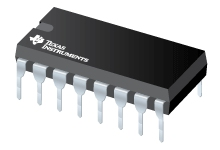CD4585B 4-Bit Magnitude Comparators | TI.com

# CD4585B (ACTIVE) 4-Bit Magnitude ComparatorsDatasheet

• Description
• Features
• Diagram
• Parametrics
• Complete your design

### Other devices and data sheet

Other device variants can also be found in the  CD4585B product page

This data sheet applies to both  CD4585B and  CD4585B-MIL

## Description

CD4585B is a 4-bit magnitude comparator designed for use in computer and logic applications that require the comparison of two 4-bit words. This logic circuit determines whether one 4-bit word (Binary or BCD) is "less than", "equal to", or "greater than" a second 4-bit word.

The CD4585B has eight comparing inputs (A3, B3, through A0, B0), three outputs (A<B,A = B,A>B) and three cascading inputs (A<B,A=B,A>B) that permit systems designers to expand the comparator function to 8, 12, 16.....4N bits. When a single CD4585B is used, the cascading inputs are connected as follows: (A<B) = low, (A=B) = high, (A>B) = high.

Cascading these units for comparison of more than 4 bits is accomplished as shown in Fig. 13.

The CD4585B types are supplied in 16-lead hermetic dual-in-line ceramic packages (F3A suffix), 16-lead dual-in-line plastic packages (E suffix), 16-lead small-outline packages (NSR suffix), and 16-lead thin shrink small-outline packages (PW and PWR suffixes).

## Features

• Expansion to 8, 12, 16.....4N bits by cascading units
• Medium-speed operation:
compares two 4-bit words in 180 ns (typ.) at 10 V
• 100% tested for quiescent current at 20 V
• Standardized symmetrical output characteristics
• 5-V, 10-V, and 15-V parametric ratings
• Maximum input current of 1 µA at 18 V over full package temperature range; 100 nA at 18 V and 25°C
• Noise margin (full package temperature range) =
1 V at VDD = 5 V
2 V at VDD = 10 V
2.5 V at VDD = 15 V
• Meets all requirements of JEDEC Tentative Standard No. 13B, "Standard Specifications for Description of 'B' Series CMOS Devices"
• Applications:
• Servo motor controls
• Process controllers